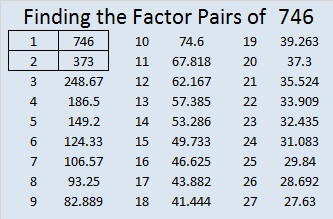# 746 and Level 1

• 746 is a composite number.
• Prime factorization: 746 = 2 x 373
• The exponents in the prime factorization are 1 and 1. Adding one to each and multiplying we get (1 + 1)(1 + 1) = 2 x 2 = 4. Therefore 746 has exactly 4 factors.
• Factors of 746: 1, 2, 373, 746
• Factor pairs: 746 = 1 x 746 or 2 x 373
• 746 has no square factors that allow its square root to be simplified. √746 ≈ 27.313000567.Here’s today’s puzzle:Print the puzzles or type the solution on this excel file: 10 Factors 2016-01-18

—————————————

Here’s a little more about the number 746:

746 is the sum of 4 consecutive numbers: 185 + 186 + 187 + 188 = 746.

746 is the hypotenuse of a Pythagorean triple triangle because 504² + 550² = 746².

746 is also the sum of three squares six different ways:

•  27² + 4² + 1² = 746
• 24² + 13² + 1² = 746
• 24² + 11² + 7² = 746
• 21² + 17² + 4² = 746
• 21² + 16² + 7² = 746
• 20² + 15² + 11² = 746

OEIS.org shared this fun number fact: 1^7 + 2^4 + 3^6 = 746.

—————————————This site uses Akismet to reduce spam. Learn how your comment data is processed.